## Example Questions

### Example Question #1 : How To Find Decimal Fractions

Simplify: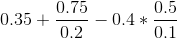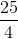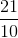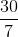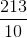Explanation:

Begin by multiplying all of your decimal fractions by: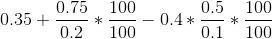Simplify: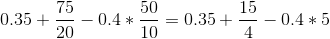Now perform the multiplication: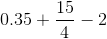The easiest thing to do next is to subtractfrom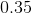: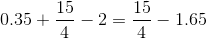Next, convert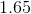into the fraction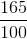: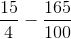Now, the common denominator can be: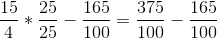Simplify: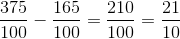### Example Question #2 : How To Find Decimal Fractions

Convert 0.825 into a fraction.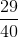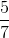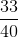0.825 in fraction form is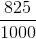.  Simplifying the fraction equals.